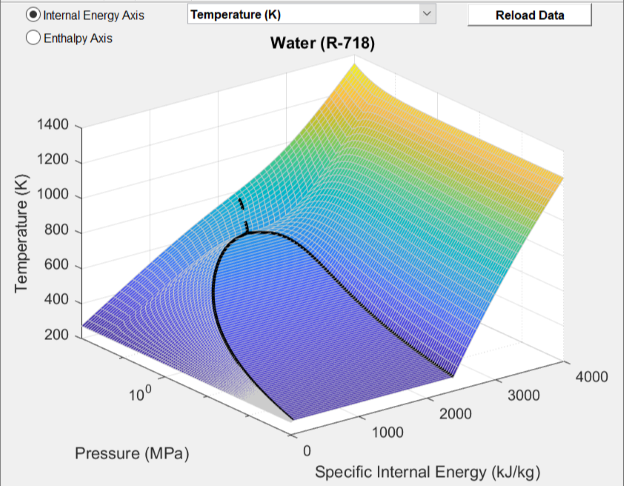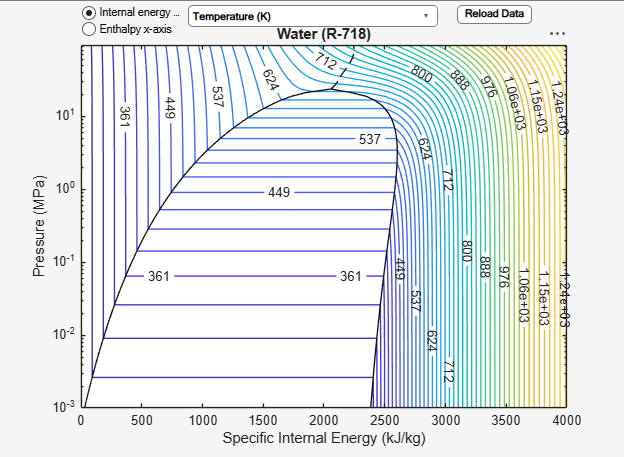# Two-Phase Fluid Predefined Properties (2P)

Predefined working fluids for two-phase networks

• Library:
• Simscape / Fluids / Two-Phase Fluid / Utilities

•## Description

The Two-Phase Fluid Predefined Properties (2P) block sets the working fluid properties of your two-phase system. The available predefined fluids are:

• Water (R-718)

• R-134a

• R-1234yf

• R-404a

• R-410a

• R-407c

• R-22

• Ammonia (R-717)

• CO2 (R-744)

• Isobutane (R-600a)

If you would like to specify your own working fluid properties, use the Foundation Library Two-Phase Fluid Properties (2P) block. If you do not specify a fluid, the system defaults will apply. See Specify Fluid Properties for more details.

### Fluid Properties Range

Water (R-718)

The fluid properties are provided for a pressure range of 0.001 to 95 MPa, and temperature range (at 1 atmosphere) of 273.16 to 1,245.8 K.

R-134a

The fluid properties are provided for a pressure range of 0.001 to 5.5 MPa, and temperature range (at 1 atmosphere) of 177.08 to 424.4 K.

R-1234yf

The fluid properties are provided for a pressure range of 0.04 to 3.2 MPa, and temperature range (at 1 atmosphere) of 223.52 to 387.45 K.

R-404a

The fluid properties are provided for a pressure range of 0.003 to 12 MPa, and temperature range (at 1 atmosphere) of 171.6 to 550.94 K.

R-410a

The fluid properties are provided for a pressure range of 0.001 to 6 MPa, and temperature range (at 1 atmosphere) of 146.19 to 427.25 K.

R-407c

The fluid properties are provided for a pressure range of 0.001 to 6 MPa, and temperature range (at 1 atmosphere) of 158.85 to 427.92 K.

R-22

The fluid properties are provided for a pressure range of 0.001 to 8 MPa, and temperature range (at 1 atmosphere) of 120.77 to 519.49 K.

Ammonia (R-717)

The fluid properties are provided for a pressure range of 0.008 to 95 MPa, and temperature range (at 1 atmosphere) of 195.5 to 543.9 K.

CO2 (R-744)

The fluid properties are provided for a pressure range of 0.55 to 50 MPa, and temperature range (at 1 MPa) of 216.94 to 1,389.4 K. Note that the triple point of CO2 is higher than atmospheric pressure, and therefore the minimum valid pressure is adjusted accordingly. When using CO2 as the working fluid, ensure that connected blocks with initial pressure parameters, such as a Reservoir (2P) block, are equal to or greater this minimum pressure.

Isobutane (R-600a)

The fluid properties are provided for a pressure range of 0.0001 to 15 MPa, and temperature range (at 1 atmosphere) of 121.02 to 516.64 K.

### Visualizing Fluid Properties

To visualize the:

• Specific volume

• Specific entropy

• Temperature

• Kinematic viscosity

• Thermal conductivity

• Prandtl number

of the network fluid as a function of specific internal energy or specific enthalpy and pressure, right-click on the block and select Fluids > Plot Fluid Properties (3D):or Fluids > Plot Fluid Properties (Contours):Use the button to regenerate the plot whenever the fluid selection or fluid parameters change.

## Ports

### Conserving

expand all

Two-phase fluid conserving port that connects the block to the network. This port connects to any point on a two-phase connection line within a block diagram. When you connect the Two-Phase Fluid Predefined Properties (2P) block to a network line, the fluid properties are propagated to all blocks in the circuit.

## Parameters

expand all

Predefined working fluid options.

Environmental pressure of the system.

Sets region in which numerical smoothing is applied. A transition region is defined around 0 MPa between the positive and negative values of the Dynamic pressure threshold for flow reversal. Within this transition region, the calculated properties are adjusted to ensure a smooth transition.

## Version History

Introduced in R2020b